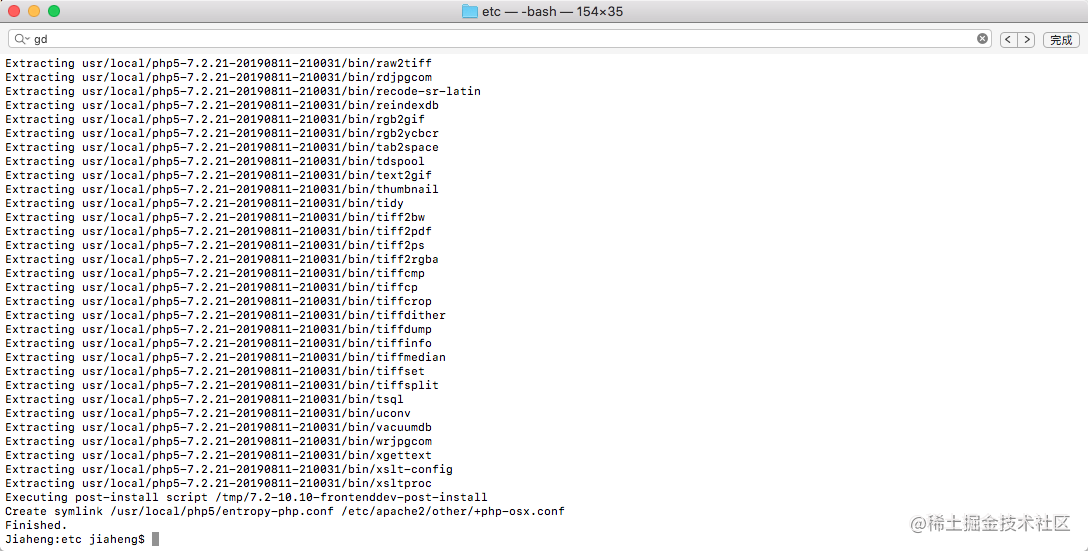# PHP>5.3版本部分新功能

+关注继续查看

【PHP5.3的新增、改进】匿名函数

1. $func = function ($arg) {
2.     print $arg; 3. }; 4.$func("Hello World");
匿名函数还可以用 use 关键字来捕捉外部变量：
1. function arrayPlus($array,$num)
2. {
3.     array_walk($array, function (&$v) use ($num) { 4.$v += $num; 5. }); 6. } 上面的代码定义了一个 arrayPlus() 函数(这不是匿名函数), 它会将一个数组($array)中的每一项，加上一个指定的数字($num).在 arrayPlus() 的实现中，我们使用了 array_walk() 函数，它会为一个数组的每一项执行一个回调函数，即我们定义的匿名函数。在匿名函数的参数列表后，我们用 use 关键字将匿名函数外的$num 捕捉到了函数内，以便知道到底应该加上多少。

1. <?php
2. class ParentBase
3. {
4.     static $property = 'Parent Value'; 5. 6. public static function render() 7. { 8. return self::$property;
9.     }
10.
11. }
12.
13. class Descendant extends ParentBase
14. {
15.     static $property = 'Descendant Value'; 16. } 17. 18. //output: Parent Value 19. echo Descendant::render(); 在这个例子中，render()方法中使用了self关键字，这是指ParentBase类而不是指Descendant类。在 ParentBase::render()方法中没法访问$property的最终值。为了解决这个问题，需要在子类中重写render()方法。通过引入延迟静态绑定功能，可以使用static作用域关键字访问类的属性或者方法的最终值
1. <?php
2.
3. class ParentBase
4. {
5.     static $property = 'Parent Value'; 6. 7. public static function render() 8. { 9. return static::$property;
10.     }
11.
12.     public static function status()
13.     {
14.         static::getStatus();
15.     }
16.
17.     protected static function getStatus()
18.     {
19.         echo "Person is alive";
20.     }
21. }
22.
23. class Descendant extends ParentBase
24. {
25.     static $property = 'Descendant Value'; 26. } 27. 28. //output: Descendant Value 29. echo Descendant::render(); PHP的面向对象体系中，提供了若干“魔术方法”，用于实现类似其他语言中的“重载”，如在访问不存在的属性、方法时触发某个魔术方法。 __call($funcname, $arguments) __callStatic($funcname, $arguments) 参数说明：$funcname String 调用的不存在的方法名称。

$arguments Array 调用方法时所带的参数。 __invoke魔术方法会在将一个对象作为函数调用时被调用： 1. class A 2. { 3. public function __invoke($args)
4.     {
5.         print "A::__invoke(): {$args}"; 6. } 7. } 8. 9.$a = new A;
10. //output: A::__invoke(): Hello World
11. $a("Hello World"); __callStatic则会在调用一个不存在的静态方法时被调用,有了__callStatic，可以省不少代码了。而且这个方法支持在子类中调用，配合上get_called_class，子类也一起魔术了 1. <?php 2. 3. class ActiveRecordBase 4. { 5. /** As of PHP 5.3.0 */ 6. public static function __callStatic($func, $arguments) 7. { 8. if ($func == 'getById') {
9.             $id =$arguments;
10.             return get_called_class() . '(' . $id . ')'; 11. } 12. 13. throw new Exception('Invalid method : ' .$name);
14.     }
15. }
16.
17. class Person extends ActiveRecordBase
18. {
19.
20. }
21.
22. // output: Person(123)
23. echo Person::getById(123);

__call 当要调用的方法不存在或权限不足时，会自动调用__call 方法。

1. <?php
2.
3. class Db
4. {
5.     private $sql = array( 6. "field" => "", 7. "where" => "", 8. "order" => "", 9. "limit" => "", 10. "group" => "", 11. "having" => "", 12. ); 13. 14. // 连贯操作调用field() where() order() limit() group() having()方法，组合sql语句 15. function __call($methodName, $args) 16. { 17. // 将第一个参数(代表不存在方法的方法名称),全部转成小写方式，获取方法名称 18.$methodName = strtolower($methodName); 19. 20. // 如果调用的方法名和成员属性数组$sql下标对应上，则将第二个参数给数组中下标对应的元素
21.         if (array_key_exists($methodName,$this->sql)) {
22.             $this->sql[$methodName] = $args; 23. } else { 24. echo '调用类' . get_class($this) . '中的方法' . $methodName . '()不存在'; 25. } 26. // 返回自己对象，则可以继续调用本对象中的方法，形成连贯操作 27. return$this;
28.     }
29.
30.     // 输出连贯操作后组合的一个sql语句，是连贯操作最后的一个方法
31.     function select()
32.     {
33.         echo "SELECT {$this->sql['field']} FROM user {$this->sql['where']} {$this->sql['order']} {$this->sql['limit']} {$this->sql['group']} 34. {$this->sql['having']}";
35.     }
36. }
37.
38. $db = new Db(); 39. 40. // 连贯操作 41.$db->field('sex, count(sex)')
42.     ->where('where sex in ("男","女")')
43.     ->group('group by sex')
44.     ->having('having avg(age) > 25')
45.     ->select();
46. ?>

1. <?php
2. //创建空间Blog
3. namespace Blog;
4.
5. class Comment
6. {
7. }
8.
9. //非限定名称，表示当前Blog空间
10. //这个调用将被解析成 Blog\Comment();
11. $blog_comment = new Comment(); 12. 13. //限定名称，表示相对于Blog空间 14. //这个调用将被解析成 Blog\Article\Comment(); 15.$article_comment = new Article\Comment(); //类前面没有反斜杆\
16.
17. //完全限定名称，表示绝对于Blog空间
18. //这个调用将被解析成 Blog\Comment();
19. $article_comment = new \Blog\Comment(); //类前面有反斜杆\ 20. 21. //完全限定名称，表示绝对于Blog空间 22. //这个调用将被解析成 Blog\Article\Comment(); 23.$article_comment = new \Blog\Article\Comment(); //类前面有反斜杆\
24.
25.
26. //创建Blog的子空间Article
27. namespace Blog\Article;
28.
29. class Comment
30. {
31. }
32. ?>

1. <?php
2. namespace Blog\Article;
3.
4. class Comment
5. {
6. }
7.
8. //创建一个BBS空间（我有打算开个论坛）
9. namespace BBS;
10.
11. //导入一个命名空间
12. use Blog\Article;
13.
14. //导入命名空间后可使用限定名称调用元素
15. $article_comment = new Article\Comment(); 16. 17. //为命名空间使用别名 18. use Blog\Article as Arte; 19. 20. //使用别名代替空间名 21.$article_comment = new Arte\Comment();
22.
23. //导入一个类
24. use Blog\Article\Comment;
25.
26. //导入类后可使用非限定名称调用元素
27. $article_comment = new Comment(); 28. 29. //为类使用别名 30. use Blog\Article\Comment as Comt; 31. 32. //使用别名代替空间名 33.$article_comment = new Comt();
34. ?>
PHP5.4

1. <?php
2. //原来的数组写法
3. $arr = array("key" => "value""key2" => "value2"); 4. // 简写形式 5.$arr = ["key" => "value""key2" => "value2"];
6. ?>
Traits

1. <?php
2. trait SayWorld
3. {
4.     public $var = 'test'; 5. public function sayHello() 6. { 7. echo 'World!'; 8. } 9. } 10. 11. class MyHelloWorld 12. { 13. // 将SayWorld中的成员包含进来 14. use SayWorld; 15. } 16. 17.$xxoo = new MyHelloWorld();
18. // sayHello()函数是来自SayWorld构件的 $xxoo->var 19.$xxoo->sayHello();
Traits还有很多神奇的功能，比如包含多个Traits, 解决冲突，修改访问权限，为函数设置别名等等。

1. (new MyClass)->xxoo();
新增支持对函数返回数组的成员访问解析
1. print ;
PHP5.5
yield的一个功能就是能有效的降低迭代的内存开销,yield关键字用于当函数需要返回一个迭代器的时候, 逐个返回值.也就是说, 每当产生一个数组元素, 就通过yield关键字返回成一个, 并且函数执行暂停, 当返回的迭代器的next方法被调用的时候, 会恢复刚才函数的执行, 从上一次被yield暂停的位置开始继续执行, 到下一次遇到yield的时候, 再次返回.
1. <?php
2. function generators()
3. {
4.     for ($i = 1;$i <= 10; $i += 1) { 5. yield$i;
6.     }
7.
8. }
9.
10. foreach (generators() as $v) { 11. echo$v;
12. }
可以用 list() 在 foreach 中解析嵌套的数组：
1. $array = [ 2. , 3. , 4. ]; 5. 6. foreach ($array as list($a,$b, $c)) 7. echo "{$a} {$b} {$c}\n";
aaaPHP实现微信支付签名算法（MD5版本及HMAC-SHA256版本）
PHP实现微信支付签名算法（MD5版本及HMAC-SHA256版本）
170 092 0Linux查看和删除PHP版本
Linux查看和删除PHP版本
83 0mac下载切换不同PHP版本
mac下载切换不同PHP版本
144 0【常见问题】宝塔面板如何安装最新版本的php扩展swoole

183 0155 0phpize是用来扩展php扩展模块的，通过phpize可以建立php的外挂模块。当php编译完成后，php的bin目录下会有phpize这个脚本文件。在编译你要添加的扩展模块之前，执行phpize就可以了；
219 0letcode两数之和php版本
letcode两数之和php版本
32 0zblog实现同分类上一篇、下一篇文章的方法(php版本)
zblog实现同分类上一篇、下一篇文章的方法(php版本)
64 0103 0

PHP完全自学手册文档教程946088